Test: Discrete Time System Analysis

# Test: Discrete Time System Analysis

Test Description

## 10 Questions MCQ Test Digital Signal Processing | Test: Discrete Time System Analysis

Test: Discrete Time System Analysis for Electrical Engineering (EE) 2022 is part of Digital Signal Processing preparation. The Test: Discrete Time System Analysis questions and answers have been prepared according to the Electrical Engineering (EE) exam syllabus.The Test: Discrete Time System Analysis MCQs are made for Electrical Engineering (EE) 2022 Exam. Find important definitions, questions, notes, meanings, examples, exercises, MCQs and online tests for Test: Discrete Time System Analysis below.
Solutions of Test: Discrete Time System Analysis questions in English are available as part of our Digital Signal Processing for Electrical Engineering (EE) & Test: Discrete Time System Analysis solutions in Hindi for Digital Signal Processing course. Download more important topics, notes, lectures and mock test series for Electrical Engineering (EE) Exam by signing up for free. Attempt Test: Discrete Time System Analysis | 10 questions in 10 minutes | Mock test for Electrical Engineering (EE) preparation | Free important questions MCQ to study Digital Signal Processing for Electrical Engineering (EE) Exam | Download free PDF with solutions
 1 Crore+ students have signed up on EduRev. Have you?
Test: Discrete Time System Analysis - Question 1

### Resolve the sequence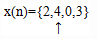into a sum of weighted impulse sequences

Detailed Solution for Test: Discrete Time System Analysis - Question 1

Explanation: We know that, x(n)δ(n-k)=x(k)δ(n-k)
x(-1)=2=2δ(n+1)
x(0)=4=4δ(n)
x(2)=3=3δ(n-2)
Therefore, x(n)= 2δ(n+1)+4δ(n)+3δ(n-2).

Test: Discrete Time System Analysis - Question 2

### The formula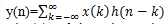​that gives the response y(n) of the LTI system as the function of the input signal x(n) and the unit sample response h(n) is known as:

Detailed Solution for Test: Discrete Time System Analysis - Question 2

Explanation: The input x(n) is convoluted with the impulse response h(n) to yield the output y(n).As we are summing the different values, we call it as Convolution sum.

Test: Discrete Time System Analysis - Question 3

### What is the order of the four operations that are needed to be done on h(k) in order to convolute x(k) and h(k)? Step-1:Folding Step-2:Multiplicaton with x(k) Step-3:Shifting Step-4:Summation

Detailed Solution for Test: Discrete Time System Analysis - Question 3

Explanation: First the signal h(k) is folded to get h(-k). Then it is shifted by n to get h(n-k). Then it is multiplied by x(k) and then summed over -∞ to ∞.

Test: Discrete Time System Analysis - Question 4

The impulse response of a LTI system is h(n)={1,1,1}. What is the response of the signal to the input x(n)={1,2,3}?

Detailed Solution for Test: Discrete Time System Analysis - Question 4

Explanation: Let y(n)=x(n)*h(n)(‘*’ symbol indicates convolution symbol)
From the formula of convolution we get,
y(0)=x(0)h(0)=1.1=1
y(1)=x(0)h(1)+x(1)h(0)=1.1+2.1=3
y(2)=x(0)h(2)+x(1)h(1)+x(2)h(0)=1.1+2.1+3.1=6
y(3)=x(1)h(2)+x(2)h(1)=2.1+3.1=5
y(4)=x(2)h(2)=3.1=3
Therefore, y(n)=x(n)*h(n)={1,3,6,5,3}.

Test: Discrete Time System Analysis - Question 5

Determine the output y(n) of a LTI system with impulse response h(n)=anu(n),|a|<1with the input sequence x(n)=u(n).

Detailed Solution for Test: Discrete Time System Analysis - Question 5

Explanation: Now fold the signal x(n) and shift it by one unit at a time and sum as follows
y(0)=x(0)h(0)=1
y(1)=h(0)x(1)+h(1)x(0)=1.1+a.1=1+a
y(2)=h(0)x(2)+h(1)x(1)+h(2)x(0)=1.1+a.1+a2.1=1+a+a2
Similarly, y(n)=1+a+a2+….an= (1-a(n+1))/(1-a).

Test: Discrete Time System Analysis - Question 6

x(n)*(h1(n)*h2(n))=(x(n)*h1(n))*h2(n)

Detailed Solution for Test: Discrete Time System Analysis - Question 6

Explanation: According to the properties of convolution, Convolution of three signals obeys Associative property.

Test: Discrete Time System Analysis - Question 7

Determine the impulse response for the cascade of two LTI systems having impulse responses h1(n)=(1/2)2 u(n) and h2(n)= (1/4)2 u(n).

Detailed Solution for Test: Discrete Time System Analysis - Question 7

Explanation: Let h2(n) be shifted and folded.
so, h(k)=h1(n)*h2(n)=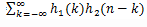For k<0, h1(n)= h2(n)=0 since the unit step function is defined only on the right hand side.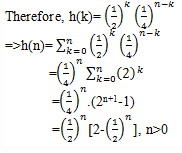Test: Discrete Time System Analysis - Question 8

x(n)*[h1(n)+h2(n)]=x(n)*h1(n)+x(n)*h2(n)

Detailed Solution for Test: Discrete Time System Analysis - Question 8

Explanation: According to the properties of the convolution, convolution exhibits distributive property.

Test: Discrete Time System Analysis - Question 9

An LTI system is said to be causal if and only if

Detailed Solution for Test: Discrete Time System Analysis - Question 9

Explanation: Let us consider a LTI system having an output at time n=n0given by the convolution formula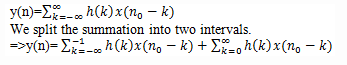=(h(0)x(n0)+h(1)x(n0-1)+h(2)x(n0-2)+….)+(h(-1)x(n0+1)+h(-2)x(n0+2)+…)
As per the definition of the causality, the output should depend only on the present and past values of the input. So, the coefficients of the terms x(n0+1), x(n0+2)…. should be equal to zero.
that is, h(n)=0 for n<0 .

Test: Discrete Time System Analysis - Question 10

x(n)*δ(n-n0)=

Detailed Solution for Test: Discrete Time System Analysis - Question 10

Explanation: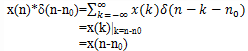## Digital Signal Processing

3 videos|50 docs|54 tests
 Use Code STAYHOME200 and get INR 200 additional OFF Use Coupon Code
Information about Test: Discrete Time System Analysis Page
In this test you can find the Exam questions for Test: Discrete Time System Analysis solved & explained in the simplest way possible. Besides giving Questions and answers for Test: Discrete Time System Analysis, EduRev gives you an ample number of Online tests for practice

## Digital Signal Processing

3 videos|50 docs|54 tests